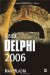Multiple if-then Condition Testing

If we have to test multiple conditions, we need to use yet another version of the if-then statement: the if-then-else-if statement. The syntax of the if-then-else-if statement looks like this:

if expression1 then   statement1 else if expression2 then   statement2 else if expression3 then   statement3;

The following application asks the user to enter a number and then writes the textual representation of the number if the user entered a number from 1 to 5.

Listing 3-5: Multiple if-then statementprogram Project1; {\$APPTYPE CONSOLE} uses   SysUtils; var   Num: Integer; begin   Write('Please enter a number: ');   ReadLn(Num);   if Num = 1 then     WriteLn('One')   else if Num = 2 then     WriteLn('Two')   else if Num = 3 then     WriteLn('Three')   else if Num = 4 then     WriteLn('Four')   else if Num = 5 then     WriteLn('Five');   ReadLn; end.The multiple if-then statement can have an else section, just like the single if-then statement. In a multiple if-then statement, the else section executes only if all previous conditions evaluate to False. The application in Listing 3-5 functions properly only if the user enters a valid number, and does absolutely nothing if the user errs. To display an error message, you can add an else section to the end of the multiple if-then statement:

if Num = 1 then   WriteLn('One') else if Num = 2 then   WriteLn('Two') else if Num = 3 then   WriteLn('Three') else if Num = 4 then   WriteLn('Four') else if Num = 5 then   WriteLn('Five') else begin   WriteLn('You''ve entered an unsupported number.');   WriteLn('Try again next year.'); end;Inside Delphi 2006 (Wordware Delphi Developers Library)
ISBN: 1598220039
EAN: 2147483647
Year: 2004
Pages: 212